# Airy disk

Airy disk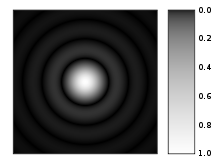Computer-generated image of an Airy disk. The gray scale intensities have been adjusted to enhance the brightness of the outer rings of the Airy pattern.Surface plot of intensity in an Airy disk.

In optics, the Airy disk (or Airy disc) and Airy pattern are descriptions of the best focused spot of light that a perfect lens with a circular aperture can make, limited by the diffraction of light.

The diffraction pattern resulting from a uniformly-illuminated circular aperture has a bright region in the center, known as the Airy disk which together with the series of concentric bright rings around is called the Airy pattern. Both are named after George Biddell Airy. The disk and rings phenomenon had been known prior to Airy; John Herschel described the appearance of a bright star seen through a telescope under high magnification for an 1828 article on light for the Encyclopedia Metropolitana:

...the star is then seen (in favourable circumstances of tranquil atmosphere, uniform temperature, &c.) as a perfectly round, well-defined planetary disc, surrounded by two, three, or more alternately dark and bright rings, which, if examined attentively, are seen to be slightly coloured at their borders. They succeed each other nearly at equal intervals round the central disc....

However, Airy wrote the first full theoretical treatment explaining the phenomenon (his 1835 "On the Diffraction of an Object-glass with Circular Aperture").

Mathematically, the diffraction pattern is characterized by the wavelength of light illuminating the circular aperture, and the aperture's size. The appearance of the diffraction pattern is additionally characterized by the sensitivity of the eye or other detector used to observe the pattern.

The most important application of this concept is in cameras and telescopes. Owing to diffraction, the smallest point to which a lens or mirror can focus a beam of light is the size of the Airy disk. Even if one were able to make a perfect lens, there is still a limit to the resolution of an image created by this lens. An optical system in which the resolution is no longer limited by imperfections in the lenses but only by diffraction is said to be diffraction limited.

The Airy disk is of importance in physics, optics, and astronomy.

## Size

Far away from the aperture, the angle at which the first minimum occurs, measured from the direction of incoming light, is given by the approximate formula:$\sin \theta \approx 1.22 \frac{\lambda}{d}$

or, for small angles, simply$\theta \approx 1.22 \frac{\lambda}{d}$

where θ is in radians and λ is the wavelength of the light and d is the diameter of the aperture. Airy wrote this as$s = \frac{2.76}{a}$

where s was the angle of first minimum in seconds of arc, a was the radius of the aperture in inches, and the wavelength of light was assumed to be 0.000022 inches (the mean of visible wavelengths). The Rayleigh criterion for barely resolving two objects that are point sources of light, such as stars seen through a telescope, is that the center of the Airy disk for the first object occurs at the first minimum of the Airy disk of the second. This means that the angular resolution of a diffraction limited system is given by the same formulae.

However, while the angle at which the first minimum occurs (which is sometimes described as the radius of the Airy disk) depends only on wavelength and aperture size, the appearance of the diffraction pattern will vary with the intensity (brightness) of the light source. Because any detector (eye, film, digital) used to observe the diffraction pattern can have an intensity threshold for detection, the full diffraction pattern may not be apparent. In astronomy, the outer rings are frequently not apparent even in a highly magnified image of a star. It may be that none of the rings are apparent, in which case the star image appears as a disk (central maximum only) rather than as a full diffraction pattern. Furthermore, fainter stars will appear as smaller disks than brighter stars, because less of their central maximum reaches the threshold of detection. While in theory all stars or other "point sources" of a given wavelength and seen through a given aperture have the same Airy disk radius characterized by the above equation (and the same size diffraction pattern), differing only in intensity (the "height" of the surface plot at upper right), the appearance is that fainter sources appear as smaller disks, and brighter sources appear as larger disks. This was described by Airy in his original work:

The rapid decrease of light in the successive rings will sufficiently explain the visibility of two or three rings with a very bright star and the non-visibility of rings with a faint star. The difference of the diameters of the central spots (or spurious disks) of different stars ... is also fully explained. Thus the radius of the spurious disk of a faint star, where light of less than half the intensity of the central light makes no impression on the eye, is determined by [s = 1.17/a], whereas the radius of the spurious disk of a bright star, where light of 1/10 the intensity of the central light is sensible, is determined by [s=1.97/a].).

Despite this feature of Airy's work, the radius of the Airy disk is often given as being simply the angle of first minimum, even in standard textbooks. In reality, the angle of first minimum is a limiting value for the size of the Airy disk, and not a definite radius.[citation needed]

## Examples

### Cameras

If two objects imaged by a camera are separated by an angle small enough that their Airy disks on the camera detector start overlapping, the objects can not be clearly separated any more in the image, and they start blurring together. Two objects are said to be just resolved when the maximum of the first Airy pattern falls on top of the first minimum of the second Airy pattern (the Rayleigh criterion).

Therefore the smallest angular separation two objects can have before they significantly blur together is given as stated above by$\sin \theta = 1.22\ \frac{\lambda}{d}$

Thus, the ability of the system to resolve detail is limited by the ratio of λ/d. The larger the aperture for a given wavelength, the finer the detail which can be distinguished in the image.

Since θ is small we can approximate this by$\frac{x}{f} = 1.22\ \frac{\lambda}{d}$

where x is the separation of the images of the two objects on the film and f is the distance from the lens to the film. If we take the distance from the lens to the film to be approximately equal to the focal length of the lens, we find$x = 1.22\ \frac{\lambda f}{d}$

but$\frac{f}{d}$ is the f-number of a lens. A typical setting for use on an overcast day would be f/8. For blue visible light, the wavelength λ is about 420 nanometers. This gives a value for x of about 4 µm. In a digital camera, making the pixels of the image sensor smaller than this would not actually increase image resolution.

### The human eye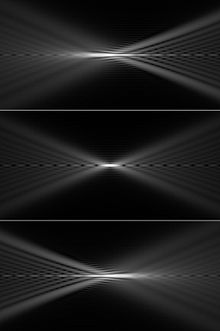Longitudinal sections through a focused beam with (top) negative, (center) zero, and (bottom) positive spherical aberration. The lens is to the left.

The fastest f-number for the human eye is about 2.1, corresponding to a diffraction-limited point spread function with approximately 1 μm diameter. However, at this f-number, spherical aberration limits visual acuity, while a 3 mm pupil diameter (f/5.7) approximates the resolution achieved by the human eye. The maximum density of cones in the human fovea is approximately 170,000 per square millimeter, which implies that the cone spacing in the human eye is about 2.5 μm, approximately the diameter of the point spread function at f/5.

### Focused laser beam

A circular laser beam with uniform intensity across the circle (a flat-top beam) focused by a lens will form an Airy disk pattern at the focus. The size of the Airy disk determines the laser intensity at the focus.

## Conditions for observation

Light from a uniformly illuminated circular aperture (or from a uniform, flattop beam) will exhibit an Airy diffraction pattern far away from the aperture due to Fraunhofer diffraction (far-field diffraction).

The conditions for being in the far field and exhibiting an Airy pattern are: the incoming light illuminating the aperture is a plane wave (no phase variation across the aperture), the intensity is constant over the area of the aperture, and the distance R from the aperture where the diffracted light is observed (the screen distance) is large compared to the aperture size, and the radius a of the aperture is not too much larger than the wavelength λ of the light. The last two conditions can be formally written as R > a2 / λ .

In practice, the conditions for uniform illumination can be met by placing the source of the illumination far from the aperture. If the conditions for far field are not met (for example if the aperture is large), the far-field Airy diffraction pattern can also be obtained on a screen much closer to the aperture by using a lens right after the aperture (or the lens itself can form the aperture). The Airy pattern will then be formed at the focus of the lens rather than at infinity.

Hence, the focal spot of a uniform circular laser beam (a flattop beam) focused by a lens will also be an Airy pattern.

In a camera or imaging system an object far away gets imaged onto the film or detector plane by the objective lens, and the far field diffraction pattern is observed at the detector. The resulting image is a convolution of the ideal image with the Airy diffraction pattern due to diffraction from the iris aperture or due to the finite size of the lens. This leads to the finite resolution of a lens system described above.

## Mathematical details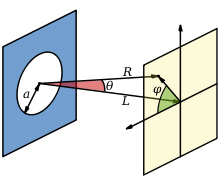Diffraction from a circular aperture. The Airy pattern is observable when R > a2 / λ (i.e. in the far field)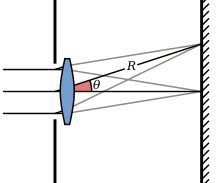Diffraction from an aperture with a lens. The far field image will (only) be formed at the screen one focal length away, where R=f (f=focal length). The observation angle θ stays the same as in the lensless case.

The intensity of the Fraunhofer diffraction pattern of a circular aperture (the Airy pattern) is given by the squared modulus of the Fourier transform of the circular aperture:$I(\theta) = I_0 \left ( \frac{2 J_1(ka \sin \theta)}{ka \sin \theta} \right )^2 = I_0 \left ( \frac{2 J_1(x)}{x} \right )^2$

where I0 is the maximum intensity of the pattern at the Airy disc center, J1 is the Bessel function of the first kind of order one, k = 2π / λ is the wavenumber, a is the radius of the aperture, and θ is the angle of observation, i.e. the angle between the axis of the circular aperture and the line between aperture center and observation point.$x = ka \sin \theta = \frac{2 \pi a}{\lambda} \frac{q}{R} = \frac{\pi q}{\lambda N}$, where q is the radial distance from the optics axis in the observation (or focal) plane and N = R / d (d=2a is the aperture diameter, R is the observation distance) is the f-number of the system.

If a lens after the aperture is used, the Airy pattern forms at the focal plane of the lens, where R = f (f is the focal length of the lens). Note that the limit for$\theta \rightarrow 0$ (or for$x \rightarrow 0$) is I(0) = I0.

The zeros of J1(x) are at$x = ka \sin \theta \approx 0, 3.8317, 7.0156, 10.1735, 13.3237, 16.4706...$. From this follows that the first dark ring in the diffraction pattern occurs where kasinθ = 3.8317..., or$\sin \theta \approx \frac{3.83}{ka} = \frac{3.83 \lambda}{2 \pi a} = 1.22 \frac{\lambda}{2a} = 1.22 \frac{\lambda}{d}$.

The radius q1 of the first dark ring on a screen is related to θ and to the f-number by$q_1 = R \sin \theta \approx 1.22 {R} \frac{\lambda}{d} = 1.22 \lambda N$

where R is the distance from the aperture, and the f-number N = R/d is the ratio of observation distance to aperture size. The half maximum of the central Airy disk (where$J_1(x)= {x} /{2 \sqrt{2}}$) occurs at x = 1.61633...; the 1/e2 point (where J1(x) = x / 2e) occurs at x = 2.58383..., and the maximum of the first ring occurs at x = 5.13562....

The intensity I0 at the center of the diffraction pattern is related to the total power P0 incident on the aperture by$I_0 = \frac{\Epsilon_A^2 A^2}{2 R^2} = \frac{P_0 A}{\lambda^2 R^2}$

where Ε is the source strength per unit area at the aperture, A is the area of the aperture (A = πa2) and R is the distance from the aperture. At the focal plane of a lens, I0 = (P0A) / (λ2f2). The intensity at the maximum of the first ring is about 1.75% of the intensity at the center of the Airy disk.

The expression for I(θ) above can be integrated to give the total power contained in the diffraction pattern within a circle of given size:$P(\theta) = P_0 [ 1 - J_0^2(ka \sin \theta) - J_1^2(ka \sin \theta) ]$

where J0 and J1 are Bessel functions. Hence the fractions of the total power contained within the first, second, and third dark rings (where J1(kasin θ) = 0) are 83.8%, 91.0%, and 93.8% respectively.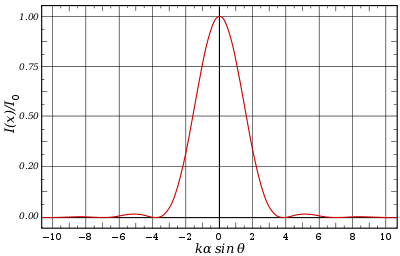The Airy Pattern on the interval kasinθ = [−10, 10]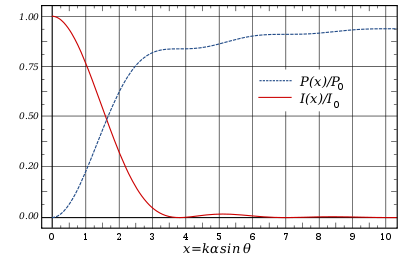The encircled power graphed next to the intensity.

## Approximation using a Gaussian profileA radial cross-section through the Airy pattern (solid curve) and its Gaussian profile approximation (dashed curve). The abscissa is given in units of the wavelength λ times the f-number of the optical system.

The Airy pattern falls rather slowly to zero with increasing distance from the center, with the outer rings containing a significant portion of the integrated intensity of the pattern. As a result, the root mean square (RMS) spotsize is undefined (i.e. infinite). An alternative measure of the spot size is to ignore the relatively small outer rings of the Airy pattern and to approximate the central lobe with a Gaussian profile, such that$I(q) \approx I'_0 \exp \left( \frac{- q^2}{2 w^2} \right) \ ,$

where I'0 is the irradiance at the center of the pattern, q represents the radial distance from the center of the pattern, and w is the Gaussian width. If we equate the peak amplitude of the Airy pattern and Gaussian profile, that is, I'0 = I0, and find the value of w giving the optimal approximation to the pattern, we obtain$w \approx 0.42 \lambda N \ ,$

where N is the f-number. If, on the other hand, we wish to enforce that the Gaussian profile has the same volume as does the Airy pattern, then this becomes$w \approx 0.44 \lambda N \ .$

In optical aberration theory, it is common to describe an imaging system as diffraction-limited if the Airy disk radius is larger than the RMS spotsize determined from geometric ray tracing (see Optical lens design). Since the RMS spotsize is equivalent to the standard deviation of a function, the Gaussian profile approximation provides a convenient means of comparison: here the RMS spotsize is just the Gaussian width parameter, w. And, using the approximation above shows that the RMS spotsize of the Gaussian approximation to the Airy disk is about one-third that of the Airy disk radius, i.e. 0.42λN as opposed to 1.22λN.

## Obscured Airy pattern

Similar equations can also be derived for the obscured Airy diffraction pattern which is the diffraction pattern from an annular aperture or beam, i.e. a uniform circular aperture (beam) obscured by a circular block at the center. This situation is relevant to many common reflector telescope designs that incorporate a secondary mirror, including Newtonian telescopes and Schmidt–Cassegrain telescopes.$I(\theta) = \frac{I_0}{ (1 - \epsilon ^2)^2} \left ( \frac{2 J_1(x)}{x} - \frac{2 \epsilon J_1(\epsilon x)}{x}\right )^2$

where$\epsilon$ is the annular aperture obscuration ratio, or the ratio of the diameter of the obscuring disk and the diameter of the aperture (beam).$\left( 0 \le \epsilon < 1 \right)$, and x is defined as above:$x=ka\sin(\theta) \approx \frac {\pi R}{\lambda N}$ where R is the radial distance in the focal plane from the optical axis, λ is the wavelength and N is the f-number of the system. The fractional encircled energy (the fraction of the total energy contained within a circle of radius R centered at the optical axis in the focal plane) is then given by:$E(R) = \frac{1}{ (1 - \epsilon ^2) } \left( 1 - J_0^2(x) - J_1^2(x) + \epsilon ^2 \left[ 1 - J_0^2 (\epsilon x) - J_1^2(\epsilon x) \right] - 4 \epsilon \int_0^x \frac {J_1(t) J_1(\epsilon t)}{t}\,dt \right)$

For$\epsilon \rightarrow 0$ the formulas reduce to the unobscured versions above.

## Comparison to Gaussian beam focus

A circular laser beam with uniform intensity profile, focused by a lens, will form an Airy pattern at the focal plane of the lens. The intensity at the center of the focus will be I0,Airy = (P0A) / (λ2f2) where P0 is the total power of the beam, A = πD2 / 4 is the area of the beam (D is the beam diameter), λ is the wavelength, and f is the focal length of the lens.

A Gaussian beam with 1 / e2 diameter of D focused through an aperture of diameter D will have a focal profile that is nearly Gaussian, and the intensity at the center of the focus will be 0.924 times I0,Airy.

## Notes and references

1. ^ Herschel, J. F. W., "Light," in Transactions Treatises on physical astronomy, light and sound contributed to the Encyclopaedia Metropolitana, Richard Griffin & Co., 1828, p. 491.
2. ^ Airy, G. B., "On the Diffraction of an Object-glass with Circular Aperture," Transactions of the Cambridge Philosophical Society, Vol. 5, 1835, p. 283-291.
3. ^ Airy, G. B., "On the Diffraction of an Object-glass with Circular Aperture," Transactions of the Cambridge Philosophical Society, Vol. 5, 1835, p. 287.
4. ^ Sidgwick, J. B., Amateur Astronomer's Handbook, Dover Publications, 1980, pg. 39-40.
5. ^ Graney, Christopher M., "Objects in Telescope Are Farther Than They Appear - How diffraction tricked Galileo into mismeasuring distances to the stars", The Physics Teacher, vol. 47, 2009, pg. 362-365.
6. ^ Airy, G. B., "On the Diffraction of an Object-glass with Circular Aperture," Transactions of the Cambridge Philosophical Society, Vol. 5, 1835, p. 288.
7. ^ Giancoli, D. C., Physics for Scientists and Engineers (3rd edition), Prentice-Hall, 2000, p. 896.
8. ^ See Sunny 16 rule.
9. ^ See cone cells for sensitivy of S cone cells.
10. ^ Hecht, Eugene (1987). Optics (2nd ed. ed.). Addison Wesley. ISBN 0-201-11609-X.  Sect. 5.7.1
11. ^ Optical System Design. McGraw-Hill Professional. 2000. ISBN 0-07-134916-2.
12. ^ "Eye Receptor Density". Archived from the original on 2008-04-30. Retrieved 2008-09-20.
13. ^ E. Hecht, Optics, Addison Wesley (2001)
14. ^ M. Born and E. Wolf, Principles of Optics (Pergamon Press, New York, 1965)
15. ^ Zhang et al., Optics Express, 15, 1543-1552 (2007): http://www.opticsinfobase.org/oe/abstract.cfm?uri=oe-15-4-1543
16. ^ Rivolta, Applied Optics, 25, 2404 (1986).
17. ^ a b Mahajan, J. Opt. Soc. Am. A, 3, 470 (1986).

Wikimedia Foundation. 2010.

### Look at other dictionaries:

• airy disk — noun Usage: usually capitalized A : the bright central spot in the system of diffraction rings formed by an optical system with light from a point source (as a star) …   Useful english dictionary

• Airy, Sir George Biddell — ▪ British astronomer born July 27, 1801, Alnwick, Northumberland, Eng. died Jan. 2, 1892, Greenwich, London       English scientist who was astronomer royal from 1835 to 1881.       Airy graduated from Trinity College, Cambridge, in 1823. He… …   Universalium

• Airy , Sir George Biddell — (1801–1892) British astronomer Airy, the son of a tax collector, was born in Alnwick in the north east of England. He attended school in Colchester before going to Cambridge University in 1819. He met with early success, producing a mathematical… …   Scientists

• disk — 1. A round, flat plate; any approximately flat circular structure. 2. SYN: lamella (2). 3. In dentistry, a circular piece of thin paper or other material, coated with an abrasive substance, used for cutting and polishing tee …   Medical dictionary

• optics — /op tiks/, n. (used with a sing. v.) the branch of physical science that deals with the properties and phenomena of both visible and invisible light and with vision. [1605 15; < ML optica < Gk optiká, n. use of neut. pl. of OPTIKÓS; see OPTIC,… …   Universalium

• Diffraction — Computer generated intensity pattern formed on a screen by diffraction from a square aperture …   Wikipedia

• Optical resolution — This article is about optical resolution in optics. For the method of separating enantiomers in chemistry, see Chiral resolution. Optical resolution describes the ability of an imaging system to resolve detail in the object that is being imaged.… …   Wikipedia

• Astronomical seeing — Schematic diagram illustrating how optical wavefronts from a distant star may be perturbed by a layer of turbulent mixing in the atmosphere. The vertical scale of the wavefronts plotted is highly exaggerated. Astronomical seeing refers to the… …   Wikipedia

• Optics — For the book by Sir Isaac Newton, see Opticks. Optical redirects here. For the musical artist, see Optical (artist). Optics includes study of dispersion of light. Optics is the branch of …   Wikipedia

• Laser beam profiler — A laser beam profiler captures, displays, and records the spatial intensity profile of a laser beam at a particular plane transverse to the beam propagation path. Since there are many types of lasers ultraviolet, visible, infrared, continuous… …   Wikipedia